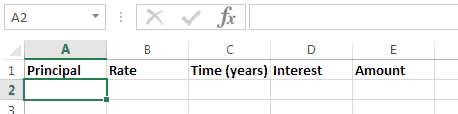### Excel formula

#### Simple interest | # gomaths.net/4222         User submitted, thanks to Simon Job

Calculate simple interest.

`=A2*B2*C2` (calculate simple interest)

`=A2+D2` (amount)

where `A2` is the Principal
`B2` is the interest rate as a percentage
`C2` is the time in years